HomeBlog

# Transformers Basics: Construction, Types, Materials and Design

Summary

The transformer is a static electrical device, mainly composed of iron core (or magnetic core) and coil. The coils have two or more windings, of which the ones connected to the power are called primary coils, and the rest are called secondary coils. Transformers are widely used in electrical equipment such as household appliances, electronic equipment, switching power supply and so on. Circuit symbols commonly used T as the beginning of the number, for example, T01, T201. This article covers the construction, functions, classification and design of transformers and materials used for building magnetic cores in transformers.

 Core Transformers Basics Purpose Ensure safe overload operation English name Transformer Category Static electrical device Function Increasing or decreasing the alternating voltages Feature 100% efficient

Catalogs

 Catalogs 1. The Composition of Transformer (3) Magnetic core parameters 5. What you should pay attention to when doing the core material selection: (4) A relatively moderate permeability (2) According to the area product (AP) method, the general steps of designing transformer are as follows: 2. The Construction and functions of Transformer (4) Coil parameters 6. Ferrite magnetic materials for switching power supply shall meet the following requirements: (5) How we choose the appropriate relative permeability? 3. High-frequency transformer design program: (5) Assembly structure (1) High saturation flux density Bs and low residual flux density Br (6) A relatively high Curie temperature (1) Core material (6) Temperature rise tests (2) Transformers or inductors are divided into three categories according to their topology: 7. Principle and method of Transformer Design (2) Magnetic core structure 4. Power transformers are divided into three categories according to their topology: (3) Low power loss at high frequency (1) There are two main ways to design transformer: Area Product(AP) Method

Introduction

1. The Composition of Transformer

• The primary side

• The secondary side

• Magnetizing inductance

• Leakage inductance

• Open-circuit or short-circuit measurement of the primary side leads to the

• magnetic inductance and the leakage inductance turns ratio respectively:

• K=Np/Ns=V1/V2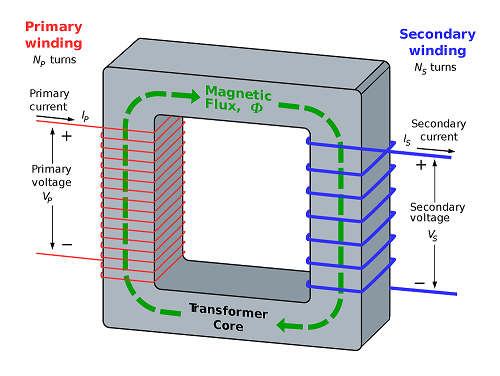2. The Construction and functions of Transformer

1. 1) Electrical isolation

2. 2) Energy storage

3. 3) Voltage change for same power input.

Visualization of how electric transformers operate

3. High-frequency transformer design program:

1. (1) Core material

2. (2) Core structure

3. (3) Core parameters

4. (4) Transformer Winding Parameter

5. (5) package assembly

6. (6) Temperature rise check

(1) Core material

Soft magnetic ferrite is widely used in switching power supply because of its own characteristics. It has the advantages of high resistivity, low AC eddy current losses, low price and easy to be machined into magnetic cores of various shapes. The disadvantages are low working magnetic flux density, low permeability, large magnetostriction and high sensitivity to temperature changes. Which kind of soft magnetic ferrite material can satisfy the design requirement of high frequency transformer more fully, only when it is carefully considered and the transformer design can reach the high cost performance.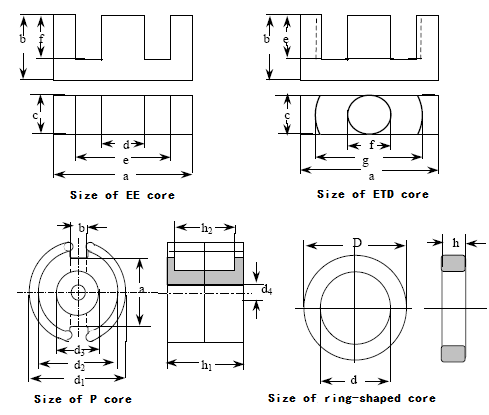(2) Magnetic core structure

The factors considered in the selection of magnetic core structure are as follows: reducing magnetic leakage and leakage inductance, increasing the area of coil heat dissipation, which is beneficial for shielding and makes it easier to wind coils, more convenient to wire for assembly and so on.

The magnetic leakage and leakage inductance are directly related to the magnetic core structure . If the magnetic core does not need air gap, then a enclosed ring-like or square type magnetic core may be used as far as possible.

(3) Magnetic core parameters

In the design of core parameters, special attention should be paid to the operating flux density only limited by the magnetization curve, but also by the losses, and also related to the working mode of power transmission. When the flux changes in one direction, there is ΔB=Bs-Br, which is not only limited by the saturation flux density, but also mainly by the losses (Losses cause temperature rise, which in turn affects magnetic flux density). The operating flux density Bm=0.6～0.7ΔB.

A air gap can decrease Br and therefore increase the flux density ΔB. The exciting current can be increased after using a air gap opening, but the core volume can be decreased either. For two-way operation of magnetic flux, the flux density ΔB is twice of the maximum operating flux density Bm, that is ΔB=2Bm. In bidirectional operating mode, we should pay attention to the problem of transformer DC magnetic bias due to the inequality of volt-second areas of positive and negative excitation variation, which is caused by different reasons. A small air gap will be needed in the core, or an DC capacitor can also be added to the circuit design.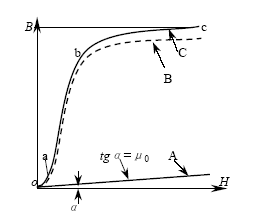Magnetic properties of ferromagnetic materials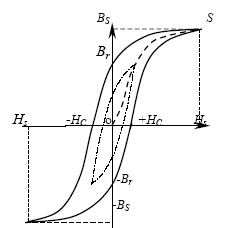Magnetic hysteresis loops of the core

(4) Coil parameters

Coil parameters include: turns, conductor section (diameter), wire form, winding arrangement and insulation. The conductor section (diameter) depends on the current density of winding, using taking 2.5～4A/mm2. When doing some choosing of section conductor diameter don’t forget to take the skin effect into consideration and do regulations necessary after some temperature rise tests of the transformer.

General winding arrangements: the primary winding is close to the core and the secondary windings & feedback windings are gradually arranged outward. The following two winding arrangements are recommended:

1) If the voltage of the original windings is high (for example, 220V) and meanwhile that of the secondary windings is low, a more appropriate arrangement is the secondary winding being close to the core, and then goes the feedback winding, the original winding is arranged on the outermost ends, which is advantageous to the insulation arrangement of the original winding to the core;

2) If we want to increase the coupling between the primary and secondary windings, we can make half of the original windings be close to the core, then goes the feedback winding and secondary winding, and the other half of the original winding being the outermost ends, which is an arrangement advantageous to reduce the leakage inductance.

(5) Assembly structure

The assembly structure of high-frequency power transformers are divided into horizontal and vertical types. If you'd like to select the planar core, sheet magnetic core and thin-film magnetic core, then a horizontal-type assembly would do you good.

(6) Temperature rise tests

The temperature rise tests can be carried out by calculation and sample test. The temperature rise is lower than the allowable temperature rise above 15 degrees, the current density and the cross section of the wire are appropriately increased. Appropriately increase the current density and decrease the cross section of the wire, and do the exact opposite if temperature rise exceeds the allowable value, such as increasing the diameter or enlarging the core if necessary, to increase the area of coil heat dissipation.

4. Power transformers are divided into three categories according to their topology:

1. (1) Flyback transformers;

2. (2) Forward transformers;

3. (3) Push-pull transformers (full-bridge/half-bridge converters)

The appropriate topologies for various core structures are shown in the following table:

 Core structure Types of converter circuit Flyback Forward Push-pull E cores + + 0 Planar E Cores - + 0 EFD Cores - + + ETD Cores 0 + + ER Cores 0 + + U Cores + 0 0 RM Cores 0 + 0 EP Cores - + 0 P Cores - + 0 Ring Cores - + +

"+"=fit; "0"=normal; "-"=unfit

Detail

5. What you should pay attention to when doing the core material selection:

(1) Soft ferrite, due to its low price, good adaptability and high performance at high frequency, has been widely used in switching power supply.

(2) Soft ferrite is commonly divided into two series: Mn-Zn ferrite and Ni-Zn ferrite. The Mn-Zn ferrite is composed of Fe2O3，MnCO3，ZnO and so on, which is widely used in all kinds of filters, inductors, transformers and so on below 1MHz. The Ni-Zn ferrite is composed of Fe2O3，NiO，ZnO and so on, which is widely used in all kinds of adjustable inductor windings, anti-jamming magnetic beads, antenna matching devices and so on above 1MHz.

(3) Mn-Zn ferrite is the most widely used core in switching power supply, and the selection of its material depends on its use. The core for the input filter part of the power supply is mostly high-conductivity magnetic core, and its material number mostly is R4K～R10K, that is, the ferrite core of relative permeability is about 4000~10000, but the main transformer and output filter are magnetic materials with high saturation flux density, where Bs is about 0.5T (5000GS).

6. Ferrite magnetic materials for switching power supply shall meet the following requirements:

(1) High saturation flux density Bs and low residual flux density Br

The residual flux density Bs has a certain influence on the transformer and winding results. Theoretically speaking, the number of turns of transformer windings can be reduced and the copper loss can be reduced because of the high Bs. In practical applications, there are different types of circuits of high frequency converters in switching power supply.

For transformers, their operations can be divided into two categories:

1) Bipolar: The circuit topologies include half bridge, full bridge, push-pull, etc. In the primary winding of the transformer, the excitation current is equal and opposite in direction during the positive and negative half cycles. Therefore, the magnetic flux changes in the magnetic core of the transformer are symmetrically moved up and down. The maximum variation range of B is  ΔB=2Bm, and the DC component of magnetic core is basically cancels out.

2) Unipolar: The circuit topologies include single-ended forward, single-ended flyback, etc. The transformer primary winding adds an unidirectional square wave pulse voltage in one cycle (this is the case for single-ended flyback). The magnetic flux density varies from the maximum Bm to the residual flux density Br in the unidirectional-excitation transformer core. If we decrease the Br and increase the saturation flux density Bs, then the △B will be increased, and the turns and copper loss will also be reduced.

(2) Transformers or inductors are divided into three categories according to their topology:

1) An DC filter inductor's magnetic core only works in one quadrant, the topologies of this operating state including Boost, Buck, buck/boost inductors, single end flyback converter transformer, forward and all push-pull converters and output filter inductors.

2) The core of transformer in the forward converter also works in one quadrant, but the transformer need to magnetic reset.

3) The core of transformer with push-pull topology is in bidirectional alternating magnetization. This kind of converters include push-pull, half-bridge and full-bridge converters, AC filter inductor and so on.

(3) Low power loss at high frequency

The power loss of ferrite not only affects the power output efficiency, but also leads to the heating of magnetic core and waveform distortion.

The heating problem of transformer is very common in practical application, which is mainly caused by copper loss and core loss of transformer. If the selected Bm is too low and the turns of winding is too many, it will cause the winding to heat up and transfer the heat to the core at the same time, and vice versa.

When selecting the ferrite material, we must make the power loss change with temperature characterized by a negative temperature coefficient. This is because if the core loss is the main heating, making the transformer temperature rise up, which then will lead to a further increase of core losses, thus it will form a vicious circle and eventually make the power tube, transformer and other components burn down. Therefore, in the researches of power ferrite at home and abroad, we must solve the problem of negative temperature coefficient of magnetic material power loss itself, which is also a remarkable feature of magnetic materials having met the requirements for power supply applications, such as PC40 from Japanese company TDK and R2KB from China manufacturers and so on.

(4) A relatively moderate permeability

(5) How we choose the appropriate relative permeability?

Well, this depends on the switching frequency of your actual circuit, mostly 2000, meanwhile its applicable frequency must be below 300kHz, and sometimes can be a little higher, but the maximum will not be higher than 500 kHz.

(6) A relatively high Curie temperature

Curie temperature is the temperature at which a magnetic material loses its magnetic properties, generally above 200 ℃. However, the actual operating temperature of the transformer should not be higher than 80℃, at which the saturation flux density Bs will drop to 70% of that at the normal temperature when the temperature is above 100℃. That is, the saturation flux density of the core will drop more seriously when the operating temperature is too high. Furthermore, when the temperature is higher than 100℃, the power loss has been experiencing a positive temperature coefficient , which will lead to a vicious circle. For R2KB2 materials, the temperature corresponding to the allowable power consumption has reached 110℃ and the Curie temperature is up to 240℃, which meets the requirements of high temperature use.

Analyses

7. Principle and method of Transformer Design

(1) There are two main ways to design transformer: Area Product (AP) Method

AP: The product of core effective cross section Ae and Area of window Aw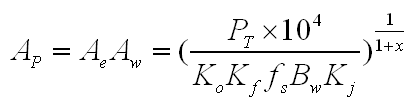• PT-The calculation power of the transformer

• Ae-Core effective cross section

• Aw- Area of window

• Ko-Core window utilization coefficient, typically 0.4

• Kf-Waveform coefficient, usually square wave being 4 and sine wave being 4.44

• Bw-The operating magnetic intensity of core

• FS-Switching frequency

• Kj-Current density coefficient, usually 395A/cm2

• X-Core structure coefficient

(2) According to the area product (AP) method, the general steps of designing transformer are as follows:

1. 1. Select the core material to calculate the apparent power of the transformer;

2. 2. Determine the core cross section AP and select the core size according to AP value;

3. 3. Calculation of the primary side inductance and the number of turns;

4. 4. Calculation of the length of air gap;

5. 5. Calculating the line diameter according to the current density and the secondary side RMS current.

6. 6. Determine whether the copper loss and iron loss meet the requirements (eg allowable loss and temperature rise)

Selecting the flyback topology, the basic parameters of the power supply are as follows:

• Input voltage: 175-264 VAC

• Output voltage: 21V

• Output current: 3A

• Output power P0=63W

• Frequency set at 60Khz

• Duty cycle set at 0.45 initially

1). Select the core material to determine the apparent power PT of the transformer;

and select the PC40 material here considering the cost factor and check the PC40 data to get Bs=0.39T, Br=0.06T.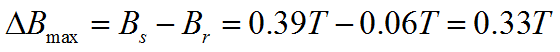In order to prevent the core from becoming saturated instantly, a certain margin is reserved. Let Bm= ΔBmax*0.6=0.198T, and pick up the 0.2T.

For flyback topology, the transformer apparent power PT is: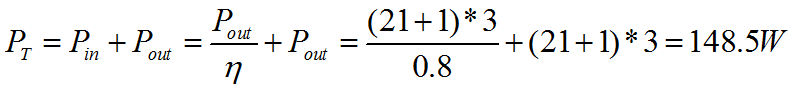2). Calculating AP values with Excel tablesWhere,

J is the current density, usually 395A/cm2, and Ku is the effective use coefficient of copper window, usually 0.2～0.4, now we set Ku as 0.4.Based on the figure above, we select the core EE3528 due to its being greater than the calculated AP value, with the following parameters:

• Ae: 84.8mm2

• AP：1.3398cm4

• Wa：158mm2

• AL：2600nH/H2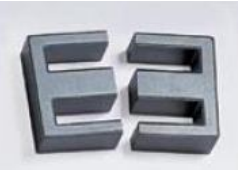In order to adapt to the abrupt load current, the power supply is designed in critical mode and the critical current is:

I0B=0.8×I0=2.4A

3) Calculation of the primary side inductance and the number of turns

(A) Minimum input voltage

• Vimin=ViACmin*1.2=210V

(B) Turns ratio

• n=[Vimin/(V0+Vf)]*[Dmax/(1-Dmax)]

• n=[210V/(21V+1V)*[0.45/(1-0.45)]

• n=7.8

(C) Peak secondary current

• ^IsB=2*IoB/(1-Dmax)

• ^IsB=2*2.4A/(1-0.45)

• ^IsB=8.72A

(D) Secondary inductance

• Ls=(V0+Vf)*(1-Dmax)*[1/(Fs*1000)]/^IsB*1000000

• Ls=(21V+1V)*(1-0.45)*[1/(60Khz*1000)]/8.72A*1000000

• Ls=23.58Uh

(E) Primary inductance

• Lp=n*n*Ls

• Lp=7.8*7.8*23.58uH

• Lp=1434uH

Primary and secondary peak currents

(F) Calculation of peak secondary current in continuous mode

• ^Isp=Io/(1-Dmax)+(^IsB/2)

• ^Isp=3A/(1-0.45)+(8.72A/2)

• ^Isp=9.81A

(G) Calculation of peak primary current in continuous mode

• ^Ipp=^Isp/n

• ^Ipp=9.8A/7.8

• ^Ipp=1.257A

(H) Calculating the turns of the primary and secondary auxiliary windings

a) Number of turns in the primary side

• Np=Lp*^Ipp/(^B*Ae)

• Np=1434uH*1.257A/(0.2*84.8)

• Np=106.28T

• After rounding:

• Np=106T

b) Number of turns in the secondary side

• Ns=Np/n

• Ns=106T/7.8

• Ns=13.58T

• After rounding:

• Ns=14T

c) Number of feedback turns

• Nv=(Vcc+Vf)/[(V0+Vf)/Ns]

• Nv=(14.5V+1V)/[(21V+1V)/14T]

• Nv=9.87T

• After rounding:

• Nv=10T

To avoid core saturation, an appropriate air gap is added to the magnetic loop, the calculation go as follows: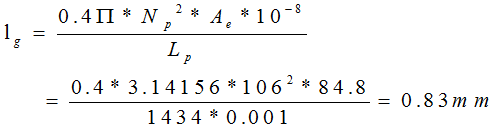The number of turns may need to be corrected by the air-gap flux edge effect.

4) There are two ways to calculate the wire diameters of the primary, secondary and auxiliary windings:

Effective current of original side diameter:

• Iprms=Po/^n/Vimin

• Iprms=63W/0.8/210V

• Iprms=0.375A

(A) Calculating the area of bare wire(B) Calculating the wire diameter (current density J to take 4A/mm2)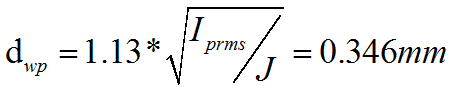Using two 0.18mm-diameter wires wound around or AWG #28 a single strand

The secondary diameter:Use four wires with a diameter of 0.25mm (AWG #31) and wind around.

Calculation of Skin Depth: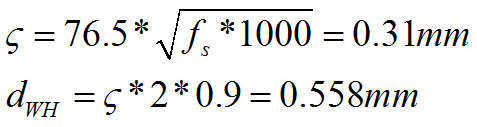The diameter of multi-strand parallel winding must be less than or equal to dwH, in single wire winding, however, if the diameter exceeds the dWH value,  the multi-strand wire winding should be taken into account.

5) Calculation of copper loss Pcu and iron loss Pfe (total transformer loss Ploss)

(A) Calculating the loss of primary and secondary windings.

Where,

MLT is the average turn length of magnetic core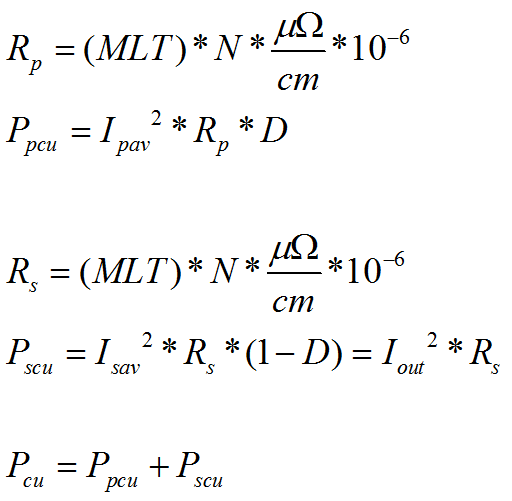(B) Calculating the allowable total loss Ploss and allowable iron loss at efficiency η

(C) According to the loss curve of iron core, the actual loss (iron loss per unit weight and actual iron loss) is obtained by: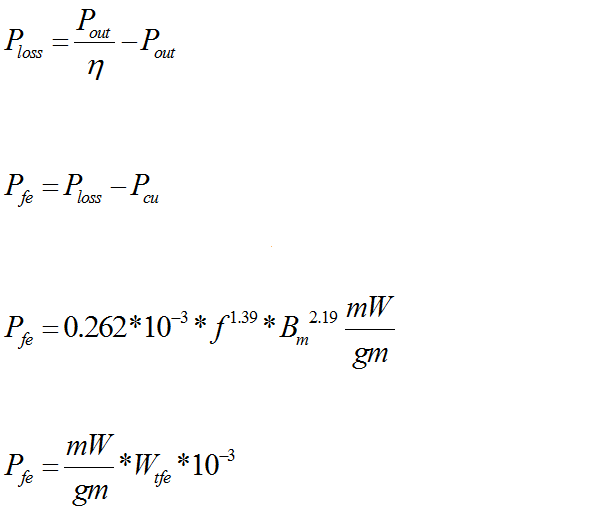The Ploss is the loss of the whole circuit, including diode/MOSFET losses and other losses, the actual losses Pfe must be much smaller than the calculated one, so here is only for reference.

(D) Calculating the loss per unit area by Φ=Ploss/As

If the temperature rise caused by Φ is less than 25 degrees, then the design is wonderful.

6) Calculating the BW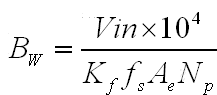The working flux density BW should be below Bs-Br within the design specifications, that is Bw<Bs-Br, to avoid saturation of the core.

Book Suggestions

Transformer Design Principles, Third EditionAug 18, 2017

In the newest edition, the reader will learn the basics of transformer design, starting from fundamental principles and ending with advanced model simulations. The electrical, mechanical, and thermal considerations that go into the design of a transformer are discussed with useful design formulas, which are used to ensure that the transformer will operate without overheating and survive various stressful events, such as a lightning strike or a short circuit event. This new edition includes a section on how to correct the linear impedance boundary method for non-linear materials and a simpler method to calculate temperatures and flows in windings with directed flow cooling, using graph theory. It also includes a chapter on optimization with practical suggestions on achieving the lowest cost design with constraints.

by Robert M. Del Vecchio and Bertrand Poulin

Handbook of Transformer Design and ApplicationsJan 22, 1993

This second edition updates what has become a standard reference on transformer design and applications, and now includes a selection of computer solutions to many transformer circuit problems. Every chapter reflects the latest technology advances - and the section on inverter transformers has been expanded to cover more fully the increasingly important subject of power supplies.

by William M. Flanagan

Transformers: Design, Manufacturing, and Materials (Professional Engineering)Nov 17, 2004

This TATA import covers basic fundamentals and design of electrical transformers, including materials, specifications and standards. Designed as a working reference for professional engineers and technicians, it will also appeal to graduate level students and researchers. Written by a team of BEHL's top power engineers, it details transformer workings from first principles through the latest in computer automation and cutting edge design.

by Bharat Heavy Electrical Limited

Relevant information "Transformers Basics: Construction, Types, Materials and Design"

About the article "Transformers Basics: Construction, Types, Materials and Design", If you have better ideas, don't hesitate to  write your thoughts in the following comment area.

You also can find more articles about electronic semiconductor through Google search engine, or refer to the following related articles:

Analysis of Calculation Theory for Transformer Temperature Rise

Some suggestions about protecting transformers

Learn Some Basic Knowledge about Capacitor Voltage TransformerHOW TO BUY ELECTRONIC COMPONENTS
Search
Inquiry
Order
Track

Delivery
FedEx
UPS
DHL
TNT

Payment Terms
By PayPal
By Credit Card
By Wire Transfer
By Western Union

After-sales Service
Quality Control
Guarantee
Return & Replacement